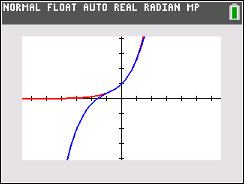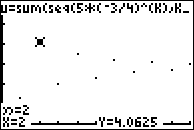### Summing Up Geometric Series

In this activity, students will explore infinite geometric series and the partial sums of geometric series. The students will determine the limits of these sequences and series using tables and graphs.
•TI-84 Plus CE
•TI-84 Plus C Silver Edition
• TI-84 Plus Silver Edition
• TI-84 Plus### Taylor Polynomials

Students learn to define a Taylor polynomial approximation to a function f of degree n about a point x = a. They also learn to graph convergence of Taylor polynomials. They use Taylor polynomials to approximate function values.
•TI-84 Plus CE
•TI-84 Plus C Silver Edition
• TI-84 Plus Silver Edition
• TI-84 Plus••